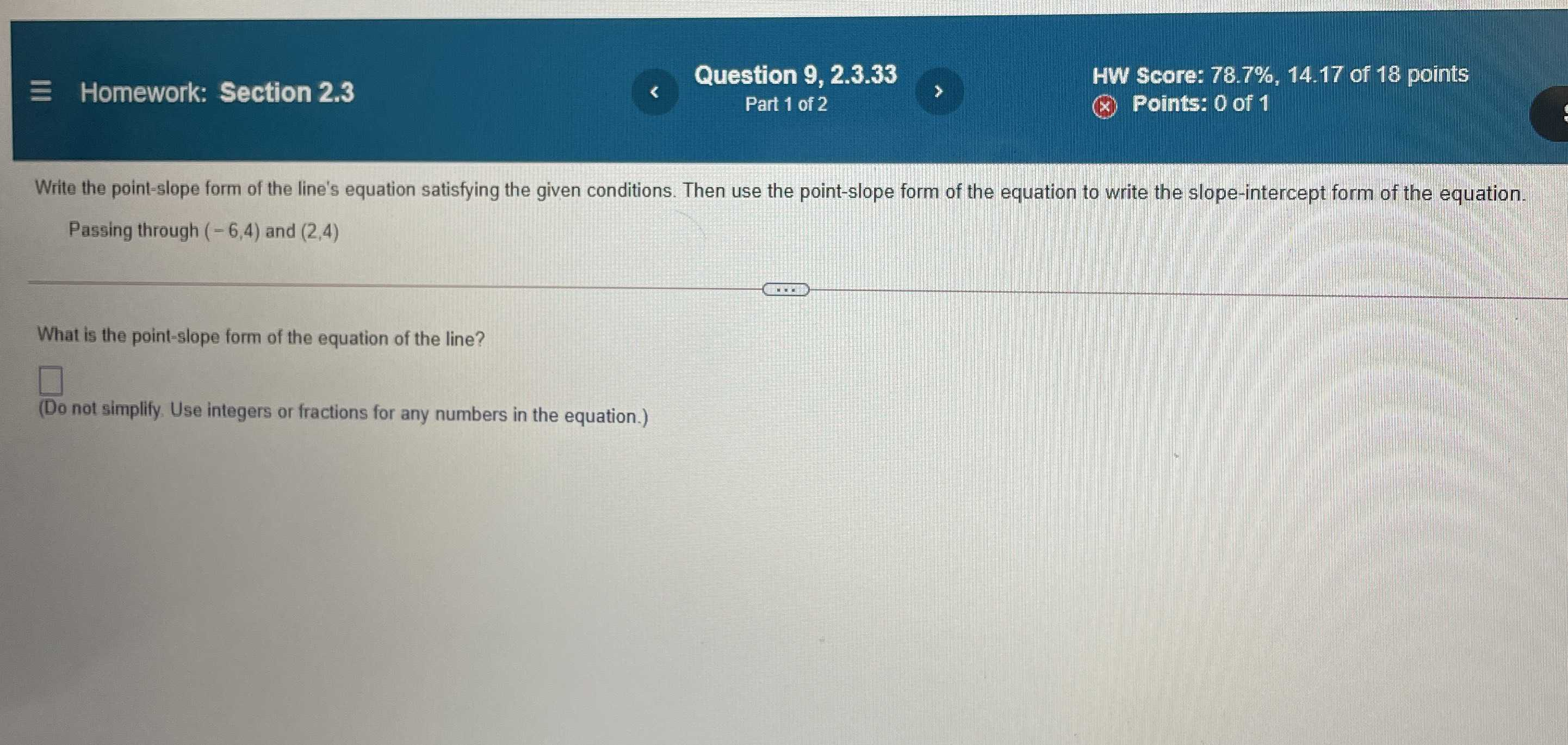### Still have math questions?

Algebra
QuestionWhe Homew Section $$2.3$$ Write the point-slope form of the line's equation satisfying the given conditions. Then use the point-slope form of the equation to write the slope-intercept form of the equation. Passing through $$( - 6,4 )$$ and $$( 2,4 )$$

What is the point-slope form of the equation of the line?

$$\square$$

(Do not simplify. Use integers or fractions for any numbers in the equation.)